Sorry, you do not have permission to ask a question, You must login to ask question. Become VIP Member

Do you need to remove the ads? Become VIP Member

Proportioning of concrete is the process of selecting quantity of cement, sand, coarse aggregate and water in concrete to obtain desired strength and quality.

The proportions of coarse aggregate, cement and water should be such that the resulting concrete has the following properties:

1. When concrete is fresh, it should have enough workability so that it can be placed in the formwork economically.
2. The concrete must possess maximum density or in the other words, it should be strongest and most water-tight.
3. The cost of materials and labour required to form concrete should be minimum.

The determination of the proportions of cement, aggregates and water to obtain the required strengths shall be made as follows:

a) By designing the concrete mix, such concrete shall be called design mix concrete, or

b) By adopting nominal mix, such concrete shall be called nominal mix concrete.

• Design mix concrete is preferred to nominal mix.
• Concrete of each grade shall be analysed separately to determine its standard deviation.

Standard Deviation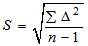Where,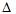= deviation of the individual test strength from the average strength of n samples.

n = Number of sample test results.

## Methods of Proportioning Concrete

### Arbitrary Method of Proportioning Concrete

The general expression for the proportions of cement, sand and coarse aggregate is 1 : n : 2n by volume.

1 : 1 : 2 and 1 : 1.2 : 2.4 for very high strength.

1 : 1.5 : 3 and 1 : 2 : 4 for normal works.

1 : 3 : 6 and 1 : 4 : 8 for foundations and mass concrete works.

#### Recommended Mixes of Concrete

The concrete as per IS 456: 2000, the grades of concrete lower than M20 are not to be used in RCC work.

 M10 1 : 3 : 6 M15 1 : 2 : 4 M20 1 : 1.5 : 3 M25 1 : 1 : 2

### Fineness Modulus Method of Proportioning Concrete

The term fineness modulus is used to indicate an index number which is roughly proportional to the average size of the particle in the entire quantity of aggregates.

The fineness modulus is obtained by adding the percentage of weight of the material retained on the following sieve and divided by 100.

The coarser the aggregates, the higher the fineness modulus.

All aggregates : 80 mm, 40 mm, 20 mm, 10 mm, and Nos. 480, 240, 120, 60, 30 and 15.

Coarse aggregates : mm, 40 mm, 20 mm, 10 mm, and No. 480.

Fine aggregates : Nos. 480, 240, 120, 60, 30 and 15.

Proportion of the fine aggregate to the combined aggregate by weight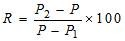Where, P = desired fineness modulus for a concrete mix of fine and coarse aggregates.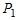= fineness modulus of fine aggregate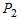= fineness modulus of coarse aggregate.

### Minimum Void Method

(Does not give satisfactory result)

The quantity of sand used should be such that it completely fills the voids of coarse aggregate. Similarly, the quantity of cement used shown such that it fills the voids of sand, so that a dense mix the minimum voids is obtained.

In actual practice, the quantity of fine aggregate used in the mix is about 10% more than the voids in the coarse aggregate and the quantity of cement is kept as about 15% more than the voids in the fine aggregate.

### Maximum Density Method:

(Not very Popular)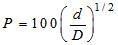Where, D = maximum size of aggregate (i.e. coarse aggregate)

P = percentage of material finer than diameter d (by weight)

d = maximum size of fine aggregate.

A box is filled with varying proportions of fine and coarse aggregates. The proportion which gives heaviest weight is then adopted.

### Water – Cement Ratio Method of Proportioning Concrete

According to the water – cement ratio law given by Abram as a result of many experiments, the strength of well compacted concrete with good workability is dependent only on the ratio.

• The lower water content produces stiff paste having greater binding property and hence the lowering the water-cement ratio within certain limits results in the increased strength.
• Similarly, the higher water content increases the workability, but lower the strength of concrete.
• The optimum water-cement ratio for the concrete of required compressive strength is decided from graphs and expressions developed from various experiments.
• Amount of water less than the optimum water decreases the strength and about 10% less may be insufficient to ensure complete setting of cement. An increase of 10% above the optimum may decrease the strength approximately by 15% while an increase in 50% may decrease the strength to one-half.
• According to Abram’s Law water-cement law, lesser the water-cement ratio in a workable mix greater will be the strength.
• If water cement ratio is less than 0.4 to 0.5, complete hydration will not be secured.

Some practical values of water cement ratio for structure reinforced concrete

• 0.45 for 1 : 1 : 2 concrete
• 0.5 for 1 : 1.5 : 3 concrete
• 0.5 to 0.6 for 1 : 2 : 4 concrete.

Concrete vibrated by efficient mechanical vibrators require less water cement ratio, and hence have more strength.

Thumb Rules for deciding the quantity of water in concrete:

(i) Weight of water = 28% of the weight of cement + 4% of the weight of total aggregate

(ii) Weight of water = 30% of the weight of cement + 5% of the weight of total aggregate

## How to Choose Water Pumps for Construction Sites?

1.25 liter in a bag of cement for M:20 concrete
It's a thumb rule and water cement ratio is 50kg cement (1 bag) contains 25 liter water; it means 1kg cement = 25/50 = 0.5

• Share
2.Nice Peter

• Share
3.Short, Simple and everything is to the point.
Thank You For sharing such useful information.

• Share
4.ok

• Share
5.Does RMC typically have lower strength than a similar grade nominal mix? Theoretically it should as it has a higher water content to ensure better workability. Or is that somehow factored in at Design Stage. Say for instance balancing the fineness of coarse aggregates.

• Share
6.Its a design grade and proportions may differ according to the quality of coarse and fine aggregates used.

• Share
7.Mass concrete is not a RCC work but a PCC work. So sections for mass concrete is huge. this counter acts the grade of concrete.

• Share
8.sir, how do you say that the proportion 1:3:6 and 1:4:8 having higher strength?

• Share
9. better to give details for Gr 20 conc. Cement –Kg, sand –Kg, Agg.—Kg like that for Gr20 to Gr 50 concrete

• Share
10. need junior site engineer (civil) for karjat site pick up from neral/karjat
call 09810168075

• Share
11.Thanks for suitable information.
B.Panya Naik
EE SPD HABTECH WARANGAL

• Share
12. I want some guide lines on the issue that is there any difference in compressive strength of concrete M15 (20 MSA )and M15 (40MSA).

• Share
13.Important information, Thanks.

• Share
14.Thanks for info…

• Share
15. why 1:3:6 and 1:4:8 proportion is used for mass concrete works? because mass concrete works require higher strength.

• Share
16. why 1:3:6 and 1:4:8 proportion is used for mass concrete works? because mass concrete works require higher strength.

• Share
17.18.